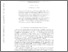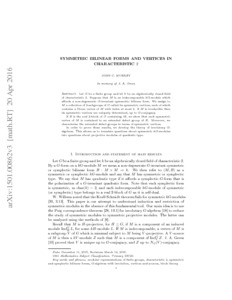# Symmetric Bilinear Forms and Vertices in Characteristic 2

Murray, John (2016) Symmetric Bilinear Forms and Vertices in Characteristic 2. Working Paper. arXiv.Previewmore...Add this article to your Mendeley library

## Abstract

Let G be a finite group and let k be an algebraically closed field of characteristic 2. Suppose that M is an indecomposable kG-module which affords a non-degenerate G-invariant symmetric bilinear form. We assign to M a collection of 2-subgroups of G called its symmetric vertices, each of which contains a Green vertex of M with index at most 2. If M is irreducible then its symmetric vertices are uniquely determined, up to G-conjugacy. If B is the real 2-block of G containing M, we show that each symmetric vertex of M is contained in an extended defect group of B. Moreover, we characterise the extended defect groups in terms of symmetric vertices. In order to prove these results, we develop the theory of involutary G-algebras. This allows us to translate questions about symmetric kG-modules into questions about projective modules of quadratic type.

Item Type: Monograph (Working Paper) Cite as: arXiv:1501.00862 Symmetric Bilinear Forms; Vertices; Faculty of Science and Engineering > Mathematics and Statistics 9110 Dr. John Murray 03 Jan 2018 15:56 arXivItem control page